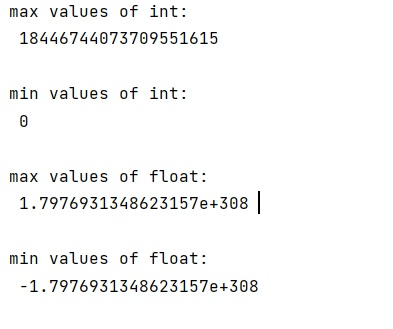# How to get the range of valid Numpy data types?

Learn how to get the range of valid Numpy data types in Python?
Submitted by Pranit Sharma, on February 10, 2023

NumPy is an abbreviated form of Numerical Python. It is used for different types of scientific operations in python. Numpy is a vast library in python which is used for almost every kind of scientific or mathematical operation. It is itself an array which is a collection of various methods and functions for processing the arrays.

## Getting the range of valid Numpy data types

In a programming language, data types are the particular format in which a value is being stored. A data type is a kind of data item, which represents what values it can take, the programming language used, or the operations that can be performed on it.

Suppose that we need to find a particular numpy type (e.g. numpy.int64, numpy.uint32, numpy.float32, etc.)

We need to find the range of all possible valid values like up to what values does numpy.int32 store.

In numpy the information about the data types can be obtained from numpy.iinfo() and numpy.finfo().

Let us understand with the help of an example,

## Python code to get the range of valid Numpy data types

```# Import numpy
import numpy as np

# Display information of different data types

print("max values of int:\n",np.iinfo(np.uint64).max,"\n")

print("min values of int:\n",np.iinfo(np.uint64).min,"\n")

print("max values of float:\n",np.finfo(np.float64).max,"\n")

print("min values of float:\n",np.finfo(np.float64).min,"\n")
```

Output: